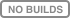Popularity
5.1
Growing
Activity
0.0
Declining
781
43
133

Programming language: C
Tags: Math

# linmath.h alternatives and similar libraries

Based on the "Math" category.
Alternatively, view linmath.h alternatives based on common mentions on social networks and blogs.

• ### GLM

OpenGL Mathematics (GLM)
• ### OpenBLAS

OpenBLAS is an optimized BLAS library based on GotoBLAS2 1.13 BSD version.
• ### ONLYOFFICE Docs — document collaboration in your environment

Powerful document editing and collaboration in your app or environment. Ultimate security, API and 30+ ready connectors, SaaS or on-premises
• ### QuantLib

The QuantLib C++ library
• ### CGal

The public CGAL repository, see the README below
• ### ceres-solver

A large scale non-linear optimization library
• ### Eigen

A high-level C++ library of template headers for linear algebra, matrix and vector operations, numerical solvers and related algorithms. [MPL2]
• ### Vc

SIMD Vector Classes for C++
• ### TinyExpr

tiny recursive descent expression parser, compiler, and evaluation engine for math expressions
• ### MIRACL

MIRACL Cryptographic SDK: Multiprecision Integer and Rational Arithmetic Cryptographic Library is a C software library that is widely regarded by developers as the gold standard open source SDK for elliptic curve cryptography (ECC).
• ### ExprTK

C++ Mathematical Expression Parsing And Evaluation Library https://www.partow.net/programming/exprtk/index.html
• ### NT2

A SIMD-optimized numerical template library that provides an interface with MATLAB-like syntax. [Boost]
• ### LibTomMath

LibTomMath is a free open source portable number theoretic multiple-precision integer library written entirely in C.
• ### Klein

P(R*_{3, 0, 1}) specialized SIMD Geometric Algebra Library
• ### muparser

muparser is a fast math parser library for C/C++ with (optional) OpenMP support.
• ### GMTL

Generic Math Template Library (forked from http://ggt.sourceforge.net/)
• ### HLSL++

Math library using hlsl syntax with SSE/NEON support
• ### Boost.Multiprecision

Boost.Multiprecision
• ### Apophenia

A C library for statistical and scientific computing
• ### safe_numerics

Replacements to standard numeric types which throw exceptions on errors
• ### blaze

high-performance C++ math library for dense and sparse arithmetic. [BSD]
• ### Wykobi

Wykobi C++ Computational Geometry Library https://www.wykobi.com
• ### Versor

[older version] Versor 1.0 C++ library for conformal geometric algebra draw routines
• ### metamath

Meta mathematics. Symbolic functions and derivatives.
• ### Xerus

A general purpose library for numerical calculations with higher order tensors, Tensor-Train Decompositions / Matrix Product States and other Tensor Networks

• ### SLIMCPP

Simple Long Integer Math for C++. Lightweight cross-platform header-only library what implements big integer arithmetic in С++17.
• ### ceval

A C/C++ library for parsing and evaluation of arithmetic expressions.

Numerical library. Complete BLAS. Hybrid cpp98/cpp11 c99/c11
• ### GMP

A C/C++ library for arbitrary precision arithmetic, operating on signed integers, rational numbers, and floating-point numbers. [LGPL3 & GPL2]

A high quality C++ linear algebra library, aiming towards a good balance between speed and ease of use. The syntax (API) is deliberately similar to Matlab. [MPL2]

Do you think we are missing an alternative of linmath.h or a related project?

### Popular Comparisons• `vec3` -- 3 element vector of floats
• `vec4` -- 4 element vector of floats (4th component used for homogenous computations)
• `mat4x4` -- 4 by 4 elements matrix, computations are done in column major order
• `quat` -- quaternion